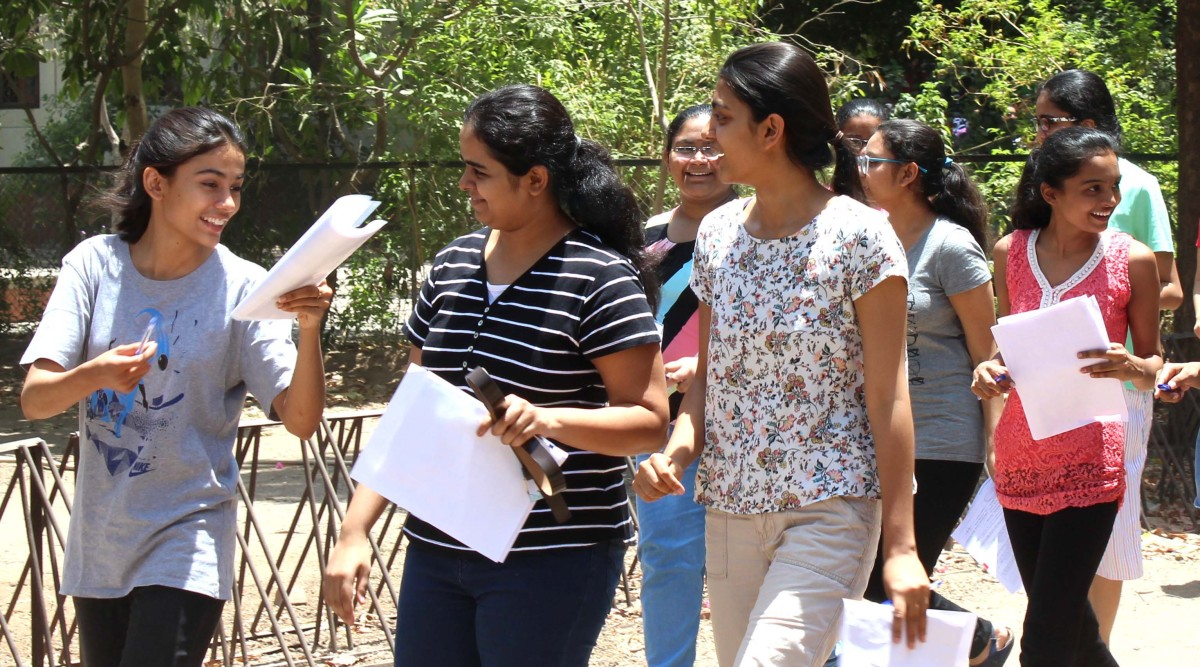3 Month's Free Subscription

# JEE Main 2022 Day 5 Session-1 paper analysis: Mathematics section trouble students

## In terms of order of difficulty – Mathematics was moderately tough while Chemistry was easy amongst the three subjects. Overall, this paper was of moderate level as per students.NEET UG is scheduled to be held on July 17 (Representative image)

On day 5 of the JEE Main 2022 session 1, the paper offered moderately difficult questions to students. As per candidates, the questions covered almost all chapters of CBSE Board class 11 and 12 syllabus. Mathematics section posed as tricky while Chemistry questions were easy to solve. .

In terms of order of difficulty – Mathematics was moderately tough while Chemistry was easy amongst the three subjects. Overall, this paper was of moderate level as per students.

In the mathematics section, questions were asked from all chapters with emphasis on Conic sections and algebra. Some questions were asked from chapters like probability, determinants, Progressions, Straight Lines and Ellipse, 3 D Geometry, Definite Integrals, Differential Equations. the numerical section had lengthy calculations. Few questions were reported as tricky.

“For a lot of students, the Mathematics part was moderate. AS anticipated, some questions involved calculations. There were 7 to 8 questions from calculus, not less than 6 questions from Algebra and 2 questions from Vectors and 3 Dimensional and about 5 questions from coordinate geometry. Regular practice and solving mock tests would be of great help in acing these papers,” Ajay Sharma, National Academic Director, Engineering at Aakash+BYJU’S said.

Subscriber Only Stories

In the Chemistry section, physical Chemistry was given more weightage compared to inorganic and organic Chemistry. Numerical based questions were mostly from Physical Chemistry. Meanwhile, Physics section was rated balanced. Numerical based questions were easy as per the students.

“As expected, numerical type questions were more in numbers than theoretical questions and almost all numerical were simple formula-based questions. Practising a sufficient number of similar tests would keep students in good stead,” Sharma said.

The second shift of the JEE Main exam began at 3 pm now and concluded at 6 pm. NTA is conducting the first session of JEE Main 2022 from June 23 to June 29.

First published on: 27-06-2022 at 01:53:58 pm
Home
ePaper
Next Story